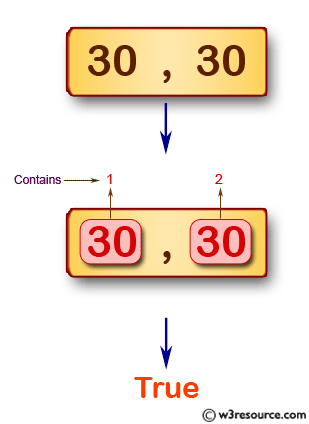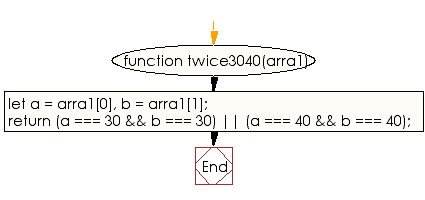# JavaScript: Test whether a given array of integers contains 30 or 40 twice

## JavaScript Basic: Exercise-79 with Solution

Write a JavaScript program to test whether a given array of integers contains 30 or 40 twice. The array length should be 0, 1, or 2.

Pictorial Presentation:Sample Solution:

HTML Code:

``````<!DOCTYPE html>
<html>
<meta charset="utf-8">
<meta name="viewport" content="width=device-width">
<title>JavaScript program to test whether a given array of integers contains 30 or 40 twice. The array length should be 0, 1, or 2.</title>
<body>

</body>
</html>
```
```

JavaScript Code:

``````function twice3040(arra1) {
let a = arra1,
b = arra1;
return (a === 30 && b === 30) || (a === 40 && b === 40);
}

console.log(twice3040([30, 30]));
console.log(twice3040([40, 40]));
console.log(twice3040([20, 20]));
console.log(twice3040());
```
```

Sample Output:

```true
true
false
true
```

Flowchart:ES6 Version:

``````function twice3040(arra1) {
let a = arra1;
let b = arra1;
return (a === 30 && b === 30) || (a === 40 && b === 40);
}

console.log(twice3040([30, 30]));
console.log(twice3040([40, 40]));
console.log(twice3040([20, 20]));
console.log(twice3040());
``````

Live Demo:

See the Pen JavaScript - Test whether an array of integers of length 2 does not contain 1 or a 3-basic-ex-78 by w3resource (@w3resource) on CodePen.

What is the difficulty level of this exercise?

Test your Programming skills with w3resource's quiz.

﻿

## JavaScript: Tips of the Day

Returns the sum of the powers of all the numbers from start to end (both inclusive)

Example:

```const sumPower = (end, power = 2, start = 1) =>
Array(end + 1 - start)
.fill(0)
.map((x, i) => (i + start) ** power)
.reduce((a, b) => a + b, 0);
console.log(sumPower(10)); // 385
console.log(sumPower(10, 3)); // 3025
console.log(sumPower(10, 3, 5)); // 2925
```

Output:

```385
3025
2925
```